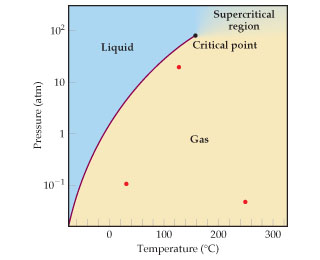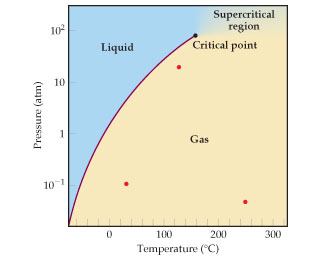# Problem: The phase diagram for SO2 is shown here.Calculate the equilibrium constant for this reaction at 100 oC.

###### FREE Expert Solution

We are being asked the equilibrium constant for the reaction at 100 oC.Recall: The equilibrium constant K is a ratio of products to reactants at a given temperature. It only deals with gaseous or aqueous compounds

80% (327 ratings)###### Problem Details

The phase diagram for SO2 is shown here.Calculate the equilibrium constant for this reaction at 100 oC.